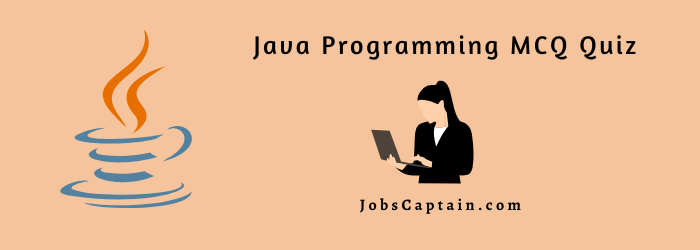# Java Programming MCQ Questions-Answer QuizYou are welcome to this quick Java MCQ Quiz. It has many basic questions on various java programming topics.

Attempt and Practice with this huge collection of Complete Java Programming MCQ questions and answers quiz. Let’s get started.

Quiz Topics

## Complete Java Programming MCQ Quiz

Question 1: Java is a ___________ language.

(A) Structured

(B) Procedural

(C) Object Oriented

(D) None

(C) Object Oriented

Question 2: Static keyword can be used on __________.

(A) Class only

(B) Methods and Classes

(C) Variables only

(D) Methods and Variables

(D) Methods and Variables

Question 3: Which of the inheritance is not directly supported in Java?

(A) Single

(B) Multiple

(C) Hierarchical

(D) Multilevel

(B) Multiple

Question 4: Re-initialization of values is not possible for variables of type ___________ .

(A) Static

(B) int

(C) float

(D) String

(A) Static

Question 5: Final keyword can be used on __________.

(A) Classes

(B) Methods

(C) Variables

(D) All the above

(D) All the above

Question 6: Constructor in java is a ____________.

(A) Special member function

(B) Keyword

(C) Garbage collector

(D) Variable

(A) Special member function

Question 7: Which constructor creates an empty string buffer with the specified capacity as length?

(A) StringBuffer()

(B) StringBuffer(String str)

(C) StringBuffer(int capacity)

(D) None of the above

(C) StringBuffer(int capacity)

Question 8: Which package contains the input/output libraries?

(A) util

(B) net

(C) io

(D) ipop

(C) io

Question 9: Which of these operators can be used to concatenate two or more String objects?

(A) =

(B) +=

(C) +

(D) .

(C) +

Question 10: Which of these method of class String is used to extract a single character from a String object?

(A) charat()

(B) charAt()

(C) CharAt()

(D) CHARAT()

(B) charAt()

Question 11: Which of these operators is used to allocate memory to array variables in Java?

(A) malloc

(B) realloc

(C) alloc

(D) new

(D) new

Question 12: Which of the following loops will execute the body of loop even when the condition controlling the loop is initially false?

(A) while

(B) do..while

(C) for

(D) if

(B) do..while

Question 13: Which of these statement is incorrect?

(A) switch statement is more efficient than a set of nested ifs.

(B) two case constants in the same switch can have identical values.

(C) switch statement can only test for equality, whereas if statement can evaluate any type of boolean expression.

(D) it is possible to create a nested switch statements.

(B) two case constants in the same switch can have identical values.

Question 14: Which of these keywords can be used in a subclass to call the constructor of superclass?

(A) super

(B) this

(C) extent

(D) extends

(A) super

Question 15: Which of these keywords can be used to prevent Method overriding?

(A) static

(B) constant

(C) protected

(D) final

(D) final

Question 16: Which of these is supported by method overriding in Java?

(A) Abstraction

(B) Encapsulation

(C) Polymorphism

(D) None of the mentioned

(C) Polymorphism

Question 17: Which of these can be overloaded?

(A) Methods

(B) Constructors

(C) Variables

(D) Methods and constructors

(D) Methods and constructors

Question 18: What is the return type of a method that does not returns any value?

(A) int

(B) void

(C) float

(D) String

(B) void

Question 19: Which of the following is not a class?

(A) String

(B) System

(C) Integer

(D) char

(D) char

Question 20: Which method can be defined only once in a program?

(A) main method

(B) finalize method

(C) static method

(D) private method

(A) main method

Question 21: Which of these statement is incorrect?

(A) Every class must contain a main() method.

(B) Applets do not require a main() method at all.

(C) There can be only one main() method in a program.

(D) main() method must be made public.

(A) Every class must contain a main() method.

Question 22: What method is used to specify a container’s layout?

(A) setLayout()

(B) setSize()

(C) area()

(D) resize()

(A) setLayout()

Question 23: Which of the following is a method having same name as that of it’s class?

(A) finalize

(B) constructor

(C) delete

(D) class

(B) constructor

Question 24: What will be the output of the program?

public class Foo { public static void main(String[] args)

{ try{return; } finally { System.out.println( “Finally” ); } } }

(A) An exception is thrown at run-time.

(B) The code runs with no output.

(C) Finally

(D) Compilation fails.

(C) Finally

Question 25: What will be the value of an after execution of the following statements?
int a = 23, b = 34; a = ((a < b) ? (b + a) : (b – a);

(A) 11

(B) 23

(C) 57

(D) 34

(C) 57

Question 26: Thread priority in Java is?

(A) integer

(B) float

(C) double

(D) long

(A) integer

Question 27: Container is a ____________ for component class.

(A) derived

(B) base

(C) interface

(D) package

(B) base

Question 28: Which of the following represent legal flow control statements?

(A) continue(inner);

(B) break();

(C) break;

(D) exit();

(C) break;

Question 29: A class can be converted to a thread by implementing the interface _________.

(B) Runnable

(C) Start

(D) Yield

(B) Runnable

Question 30: A class contains __________.

(A) data and variables

(B) data members and constructors

(C) data members and member function

(D) member functions